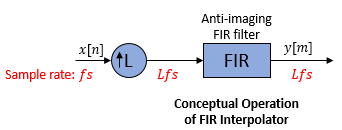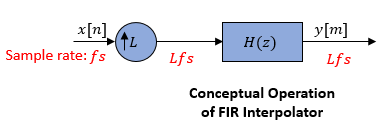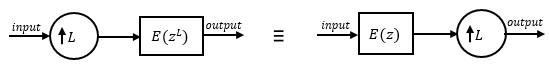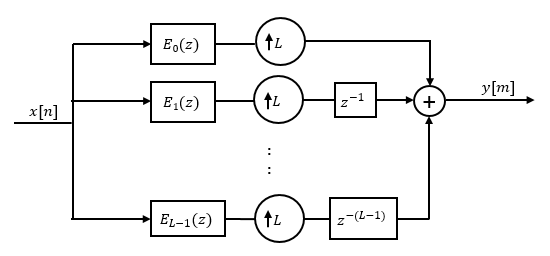# FIR Interpolation

Upsample and filter input signals

## Library

Filtering / Multirate Filters

`dspmlti4`

•## Description

The FIR Interpolation block upsamples an input by the integer upsampling factor L along the first dimension. The FIR interpolator (as shown in the schematic) conceptually consists of an upsampler followed by an FIR anti-imaging filter, which is usually an approximation of an ideal band-limited interpolation filter. To design an FIR anti-imaging filter, use the `designMultirateFIR` function.

The upsampler upsamples each channel of the input to a higher rate by inserting L–1 zeros between samples. The direct-form FIR filter that follows filters each channel of the upsampled data. The resulting discrete-time signal has a sample rate that is L times the original sample rate.Note that the actual block algorithm implements a polyphase structure, an efficient equivalent of the combined system depicted in the diagram. For more details, see Algorithms.

You can use the FIR Interpolation block inside triggered subsystems when you set the Rate options parameter to ```Enforce single-rate processing```.

Under specific conditions, this block also supports SIMD code generation. For more details, see Code Generation.

### Specifying the Filter Coefficients

To specify the filter coefficients, select the mode you want the FIR Interpolation block to operate in. Select the mode in the Coefficient source group box.

• Dialog parameters — Enter information about the filter, such as coefficients in the block dialog box.

• Input port — Specify the filter coefficients as an input to the block. Coefficient values are tunable (can change during simulation), while their properties must remain constant.

• Filter object — Specify the filter using a `dsp.FIRInterpolator` System object™.

• Auto (default) — Choose the filter coefficients of an FIR Nyquist filter, predesigned for the interpolation factor specified in the block dialog box.

When you select Dialog parameters, you use the FIR filter coefficients parameter to specify the numerator coefficients of the FIR filter transfer function H(z).

`$H\left(z\right)={b}_{0}+{b}_{1}{z}^{-1}+...+{b}_{N}{z}^{-N}$`

You can generate the FIR filter coefficient vector, b = [b0, b1, …, bN], using one of the DSP System Toolbox™ filter design functions such as `designMultirateFIR`, `firnyquist`, `firhalfband`, `firgr` or `firceqrip`.

To act as an effective anti-imaging filter, the coefficients usually correspond to a lowpass filter with a normalized cutoff frequency no greater than the reciprocal of the interpolation factor. To design such a filter, use the `designMultirateFIR` function.

The block internally initializes all filter states to zero.

When you select Auto, the block designs an FIR interpolator with the interpolation factor specified in Interpolation factor. The `designMultirateFIR` function designs the filter and returns the coefficients used by the block.

### Frame-Based Processing

When you set the Input processing parameter to `Columns as channels (frame based)`, the block resamples each column of the input over time. In this mode, the block can perform either single-rate or multirate processing. You can use the Rate options parameter to specify how the block resamples the input:

• When you set the Rate options parameter to `Enforce single-rate processing`, the input and output of the block have the same sample rate. To interpolate the output while maintaining the input sample rate, the block resamples the data in each column of the input such that the frame size of the output (Ko) is L times larger than that of the input (Ko = Ki*L).

For an example of single-rate FIR Interpolation, see Example 1 — Single-Rate Processing.

• When you set the Rate options parameter to `Allow multirate processing`, the input and output of the FIR Interpolation block are the same size. However, the sample rate of the output is L times faster than that of the input. In this mode, the block treats a Ki-by-N matrix input as N independent channels. The block interpolates each column of the input over time by keeping the frame size constant (Ki=Ko), while making the output frame period (Tfo) L times shorter than the input frame period (Tfo = Tfi/L).

See Example 2 — Multirate Frame-Based Processing for an example that uses the FIR Interpolation block in this mode.

### Sample-Based Processing

When you set the Input processing parameter to `Elements as channels (sample based)`, the block treats a P-by-Q matrix input as P*Q independent channels, and interpolates each channel over time. The output sample period (Tso) is L times shorter than the input sample period (Tso = Tsi/L), while the input and output sizes remain identical.

### Latency

When you run your models in Simulink® `SingleTasking` mode or set the Input processing parameter to ```Columns as channels (frame based)``` and the Rate options parameter to `Enforce single-rate processing`, the FIR Interpolation block always has zero-tasking latency. Zero-tasking latency means that the block propagates the first filtered input sample (received at time t=`0`) as the first output sample. That first output sample is then followed by L`1` interpolated values, the second filtered input sample, and so on.

The only time the FIR Interpolation block exhibits latency is when you set the Rate options parameter set to ```Allow multirate processing``` and run your models in Simulink `MultiTasking` mode. The amount of latency for multirate, multitasking operation depends on the setting of the Input processing parameter, as shown in the following table.

Input processingLatency

```Elements as channels (sample based)```

L samples

```Columns as channels (frame based)```

L frames (Ki samples per frame)

When the block exhibits latency, the default initial condition is zero. Alternatively, you can use the Output buffer initial conditions parameter to specify a matrix of initial conditions containing one value for each channel or a scalar initial condition to be applied to all channels. The block scales the Output buffer initial conditions by the Interpolation factor and outputs the scaled initial conditions until the first filtered input sample becomes available.

When the block is in sample-based processing mode, the block outputs the scaled initial conditions at the start of each channel, followed immediately by the first filtered input sample, then L–1 interpolated values, and so on.

When the block is in frame-based processing mode and using the default initial condition of zero, the first Ki*L output rows contain zeros, where Ki is the input frame size. The first filtered input sample (first filtered row of the input matrix) appears in the output as sample Ki*L+`1`. That value is then followed by L–1 interpolated values, the second filtered input sample, and so on.

Note

### Fixed-Point Data Types

The following diagram shows the data types used within the FIR Interpolation block for fixed-point signals.You can set the coefficient, product output, accumulator, and output data types in the block dialog box as discussed in Dialog Box section. This diagram shows that input data is stored in the input buffer with the same data type and scaling as the input. The block stores filtered data and any initial conditions in the output buffer using the output data type and scaling that you set in the block dialog box.

When at least one of the inputs to the multiplier is real, the output of the multiplier is in the product output data type. When both inputs to the multiplier are complex, the result of the multiplication is in the accumulator data type. For details on the complex multiplication performed by this block, see Multiplication Data Types.

Note

When the block input is fixed point, all internal data types are signed fixed point.

## Examples

### Example 1 — Single-Rate Processing

In the ex_firinterpolation_ref2, the FIR Interpolation block interpolates a single-channel input with a frame size of `16`. Because the block is doing single-rate processing and the Interpolation factor parameter is set to `4`, the output of the FIR Interpolation block has a frame size of `64`. As shown in the following figure, the input, and output of the FIR Interpolation block have the same sample rate.### Example 2 — Multirate Frame-Based Processing

In the ex_firinterpolation_ref1, the FIR Interpolation block interpolates a single-channel input with a frame period of 1 second (Sample time = `1/64` and Samples per frame = `64`). Because the block is doing multirate frame-based processing and the Interpolation factor parameter is set to `4`, the output of the FIR Interpolation block has a frame period of `0.25` seconds. As shown in the following figure, the input and output of the FIR Interpolation block have the same frame size, but the sample rate of the output is `1/4` times that of the input.### Example 3

The `ex_polyphaseinterp` model illustrates the underlying polyphase implementations of the FIR Interpolation block. Run the model, and view the results on the scope. The output of the FIR Interpolation block matches the output of the Polyphase Interpolation Filter block.

### Example 4

The `ex_mrf_nlp` model illustrates the use of the FIR Interpolation block in a number of multistage multirate filters.

## Dialog Box

### Coefficient Source

The FIR Interpolation block can operate in four different modes. Select the mode in the Coefficient source group box.

• Dialog parameters — Enter information about the filter, such as coefficients, in the block mask.

• Input port — Specify the filter coefficients with a Num input port. The Num input port appears when you select the Input port option. Coefficient values obtained through Num are tunable (can change during simulation), while their properties must remain constant.

• Filter object — Specify the filter using a `dsp.FIRInterpolator` System object.

• Auto (default) — Choose the coefficients of an FIR Nyquist filter, predesigned for the Interpolation factor specified in the block dialog box.

Different items appear on the FIR Interpolation block dialog box depending on whether you select Dialog parameters, Input port, Filter object, or Auto in the Coefficient source group box.

## References

 Fliege, N. J. Multirate Digital Signal Processing: Multirate Systems, Filter Banks, Wavelets. West Sussex, England: John Wiley & Sons, 1994.

 Orfanidis, Sophocles J. Introduction to Signal Processing. Upper Saddle River, NJ: Prentice-Hall, 1996.

## Supported Data Types

PortSupported Data Types

Input

• Double-precision floating point

• Single-precision floating point

• Fixed point

• 8-, 16-, and 32-bit signed integers

• 8-, 16-, and 32-bit unsigned integers

Output

• Double-precision floating point

• Single-precision floating point

• Fixed point

• 8-, 16-, and 32-bit signed integers

• 8-, 16-, and 32-bit unsigned integers

## Algorithms

The FIR interpolation filter is implemented efficiently using a polyphase structure.

To derive the polyphase structure, start with the transfer function of the FIR filter:

`$H\left(z\right)={b}_{0}+{b}_{1}{z}^{-1}+...+{b}_{N}{z}^{-N}$`

N+1 is the length of the FIR filter.

You can rearrange this equation as follows:

`$H\left(z\right)=\begin{array}{c}\left({b}_{0}+{b}_{L}{z}^{-L}+{b}_{2L}{z}^{-2L}+..+{b}_{N-L+1}{z}^{-\left(N-L+1\right)}\right)+\\ {z}^{-1}\left({b}_{1}+{b}_{L+1}{z}^{-L}+{b}_{2L+1}{z}^{-2L}+..+{b}_{N-L+2}{z}^{-\left(N-L+1\right)}\right)+\\ \begin{array}{c}⋮\\ {z}^{-\left(L-1\right)}\left({b}_{L-1}+{b}_{2L-1}{z}^{-L}+{b}_{3L-1}{z}^{-2L}+..+{b}_{N}{z}^{-\left(N-L+1\right)}\right)\end{array}\end{array}$`

L is the number of polyphase components, and its value equals the interpolation factor that you specify.

You can write this equation as:

`$H\left(z\right)={E}_{0}\left({z}^{L}\right)+{z}^{-1}{E}_{1}\left({z}^{L}\right)+...+{z}^{-\left(L-1\right)}{E}_{L-1}\left({z}^{L}\right)$`

E0(zL), E1(zL), ..., EL-1(zL) are polyphase components of the FIR filter H(z).

Conceptually, the FIR interpolation filter contains an upsampler followed by an FIR lowpass filter H(z).Replace H(z) with its polyphase representation.Here is the multirate noble identity for interpolation.Applying the noble identity for interpolation moves the upsampling operation to after the filtering operation. This move enables you to filter the signal at a lower rate.You can replace the upsampling operator, delay block, and adder with a commutator switch. The switch starts on the first branch 0 and moves in the counter clockwise direction, each time receiving one sample from each branch. The interpolator effectively outputs L samples for every one input sample it receives. Hence the sample rate at the output of the FIR interpolation filter is Lfs.## Extended Capabilities

### Fixed-Point ConversionDesign and simulate fixed-point systems using Fixed-Point Designer™.

Introduced before R2006a

## SupportGet trial now